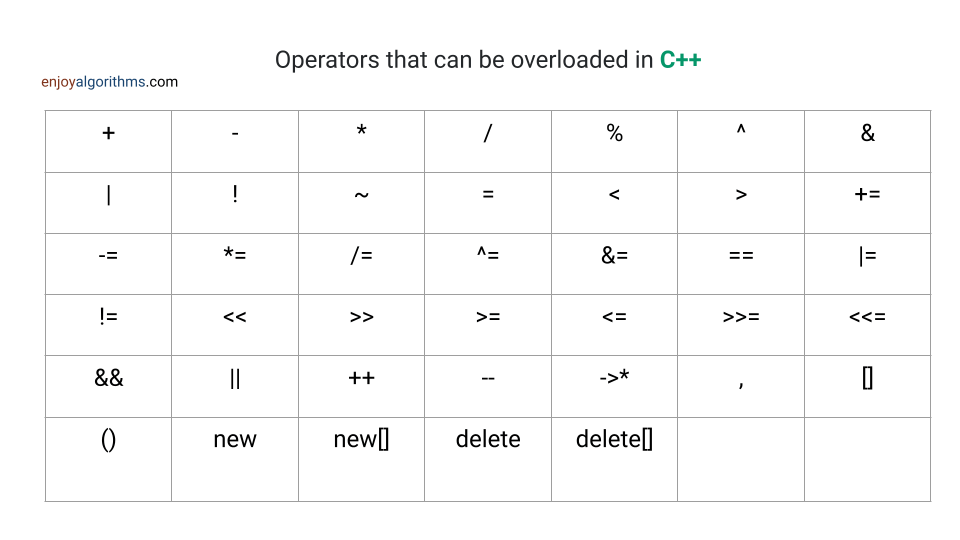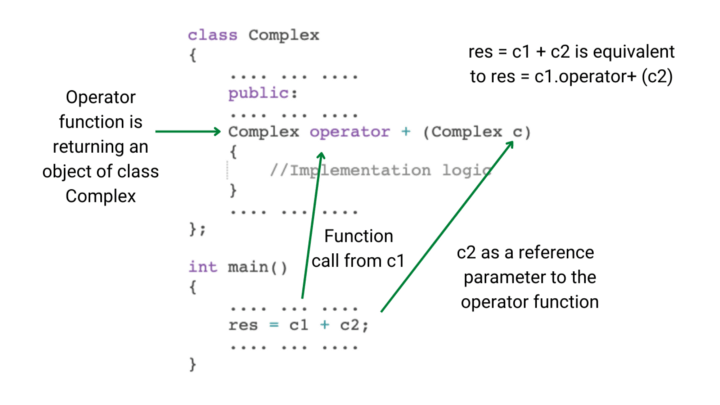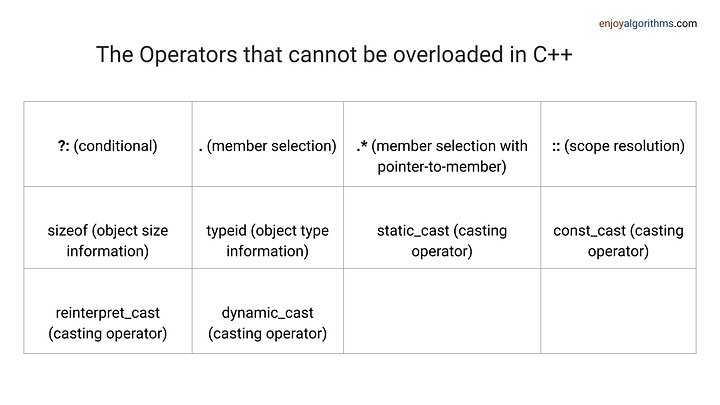Operator overloading is a feature in C++ that enables operators (such as +, -, etc.) to work with user-defined data types. This mechanism is known as compile-time polymorphism and provides the advantage of customizing operator behavior for different data types.

For example, we can overload "+" operator to perform addition on integers, concatenation on strings, and addition on complex numbers. This enhances the versatility of operators, allowing them to operate on a wider range of data types.

#### What is operator function?

An operator function is a specialized type of function that provides an alternate implementation for a particular operator. It is similar in syntax to a regular function, but its name starts with the "operator" keyword followed by the operator symbol.

We can define multiple operator functions for the same operator, which can be differentiated based on the number and type of operands they are used with. For example, the "+" operator can have a different operator function implementation for integers, strings, and complex numbers. This allows for the operator to be customized to meet specific requirements.

``````class ClassName
{
...
public
ReturnType operator OperatorSymbool(argument list)
{
// Implementation logic
}
...
};``````
• ReturnType: Type of value returned by an operator function.
• operator: Keyword used in programming languages to perform specific operations.
• OperatorSymbol: Symbol of the operator being overloaded in the program.
• argument list: List of arguments passed to a function when it is called.Suppose we don't know operator overloading and want to add two complex numbers. We can fo this by creating a class for complex numbers, called "Complex". Inside the class, we define a public method called "add", which perform the addition of two complex numbers. This method will accept two complex numbers as arguments and return the result of their addition as a new complex number.

``````class Complex {
public:
Complex add(Complex c1, Complex c2) {
// Perform addition of c1 and c2 and return the result
return result;
}
};

//This approach works.
//But it requires us to call add method
Complex c1, c2, res;

As we know operator overloading allows us to change the behavior of operators to work with user-defined data types. So overload the "+" operator for complex numbers, we can define an operator overloading function inside the "Complex" class.

This function will specify the new behavior of the "+" operator when it is used with complex numbers. The function can either be defined as a member function or a friend function of the class, depending on the specific requirements of the operator and the operands it is operating on.

After defining the operator function, we can now add two complex numbers, c1 and c2, using a simple statement: res = c1 + c2, which is equivalent to res = c1.operator+ (c2). This makes the code more intuitive and easier to understand.

#### Implementation code

``````#include <iostream>
using namespace std;

class Complex {
private:
int real, imag;
public:
Complex(int r = 0, int i = 0) {
real = r;
imag = i;
}
Complex operator + (Complex c) {
Complex temp;
temp.real = real + c.real;
temp.imag = imag + c.imag;
return temp;
}

int getReal(){
return real;
}

int getImag(){
return imag;
}
};

int main() {
Complex c1(4, 7);
Complex c2(3, 5);
Complex res;
res = c1 + c2;

cout << "Result: " << res.getReal() << " + " << res.getImag() << "i" << endl;

return 0;
}

//output Result: 7 + 12i``````We can also write the function signature as follows:

``Complex operator + (const Complex& c)``

This version has several notable differences from the previous version:

• The argument "c" is passed by reference using the "&" operator, meaning the function will receive a direct link to the original object instead of a copy.
• The argument "c" is also designated as "const", indicating that the function cannot alter the object it is passed.

These modifications are considered best practices for the following reasons:

• By passing an object by reference, the function can access the original object without creating a duplicate, making it more efficient and avoid unnecessary overhead of creating a new object.
• Designating an object as "const" helps prevent unintended modifications to the object, reducing the risk of bugs and unexpected behavior in the code.``````class opr
{
private:
int a;
float b;

public:
opr(int a, float b)
{
this->a = a;
this->b = b;
}

opr operator + (opr test)
{
opr tmp(0, 0.0);
tmp.a = a + test.a;
tmp.b = b + test.b;
return tmp;
}

void show()
{
cout << a << " " << b << '\n';
}
};

int main()
{
opr obj1(1, 3.3);
opr obj2(2, 1.5);
opr obj3;
obj3 = obj1 + obj2;
obj3.show();
return 0;
}``````

When overloading operators in C++, there are several important rules to keep in mind:

• At least one of the operands must be a user-defined data type.
• Only built-in operators can be overloaded. This means that we cannot create new operators and only existing operators can be altered to work differently.
• Overloaded operators cannot have default parameters, with the exception of an empty parameter list "()".
• Number of operands cannot be changed. For example, unary operators remain unary and binary operators remain binary.
• The assignment operator "=" is automatically overloaded by the compiler for every class. In other words, there is no need to create a separate operator function for the assignment operator. We can use it to copy objects of the same class, similar to using a copy constructor.
• When overloading operators, it's crucial to use them correctly and consistently to make the code more readable and understandable.

Unary operators are operators that operate on a single operand. The increment operator "++" and decrement operator "--" are examples of unary operators. For example, the increment operator "++" increases the value of its operand by 1. It can be used as a prefix operator (placed before the operand) or as a postfix operator (placed after the operand).

For example:

``````int x = 5;
// x is incremented to 6, and y is set to 6
int y = ++x;
// x is incremented to 7, but z is set to the original value of x (6)
int z = x++;``````

Similarly, the decrement operator "--" decreases the value of its operand by 1. It can also be used as a prefix or postfix operator. For example:

``````int x = 5;
// x is decremented to 4, and y is set to 4
int y = --x;
// x is decremented to 3, but z is set to the original value of x (4)
int z = x--;``````

#### Implementation code to overload increment operator (++)

In the following code, we have defined a class called "Value" with a single private member variable "count". We also defined a constructor function that initializes this member variable to the value 2. Inside the class, we have defined the "operator++" function as a member function, which is overloaded twice (with and without an int argument). These functions increment the value of the "count" member variable by 1.

Inside the main function, we create a "Value" object (v) and use the "++" operator on it multiple times. We then use the "getCount" method to retrieve the value of the "count" member variable.

``````class Value
{
private:
int count;
public:
Value() : count(2) {}
// prefix version of ++ operator
void operator ++ ()
{
++count;
}

// postfix version of ++ operator
void operator ++ (int)
{
++count;
}

int getCount()
{
return count;
}
};

int main()
{
Value v;
v++;
cout << v.getCount() << "\n";
++v;
++v;
cout << v.getCount() << "\n";
return 0;
}

//output
//3
//5``````

The only difference between prefix and postfix versions of a unary operator function is the argument list. The prefix version takes no arguments, while postfix version takes a single argument of type "int". This argument is not actually used to pass an integer value, but rather serves as a signal to the compiler that the function should be used to overload postfix form of the operator.

A member function is a function that is defined inside a class and acts upon objects of that class. In regards to operator overloading, unary operators (operators that operate on a single argument) have no arguments in their list, while binary operators (operators that operate on two arguments) have one argument.

``````class Complex
{
private:
double real;
double imag;

public:
Complex(double real = 0, double imag = 0) : real(real), imag(imag) {}

// overload the + operator as a member function
Complex operator + (const Complex& other) const
{
return Complex(real + other.real, imag + other.imag);
}

void print() const
{
cout << real << " + " << imag << "i" << endl;
}
};

int main()
{
Complex c1(1, 2);
Complex c2(3, 4);
Complex c3 = c1 + c2;
c3.print();
return 0;
}``````

A friend function is not a member of a class, but has direct access to the private and protected members and can be declared in either the private or public section of the class. It offers greater flexibility compared to member functions.

In other words, if the operator function needs access to the private and protected members of a class, it can be defined as a friend function. In this case, unary operators have a single argument, while binary operators have two arguments.

``````class Complex
{
private:
double real;
double imag;

public:
Complex(double real = 0, double imag = 0) : real(real), imag(imag) {}

friend Complex operator + (const Complex& c1, const Complex& c2);

void print()
{
cout << real << " + " << imag << "i" << endl;
}
};

// overload the + operator as a friend function
Complex operator + (const Complex& c1, const Complex& c2)
{
return Complex(c1.real + c2.real, c1.imag + c2.imag);
}

int main()
{
Complex c1(1, 2);
Complex c2(3, 4);
Complex c3 = c1 + c2;
c3.print();
return 0;
}``````

Non-member function is not a member of the class and does not have access to the private and protected members.

``````class Complex
{
public:
double real;
double imag;
Complex(double real = 0, double imag = 0) : real(real), imag(imag) {}

void print() const
{
cout << real << " + " << imag << "i" << endl;
}
};

// overload the + operator as a non-member function
Complex operator + (const Complex& c1, const Complex& c2)
{
return Complex(c1.real + c2.real, c1.imag + c2.imag);
}

int main()
{
Complex c1(1, 2);
Complex c2(3, 4);
Complex c3 = c1 + c2;
c3.print();
return 0;
}``````

Enjoy learning, Enjoy oops!

☆ 16-Week Live DSA Course
☆ 10-Week Live DSA Course